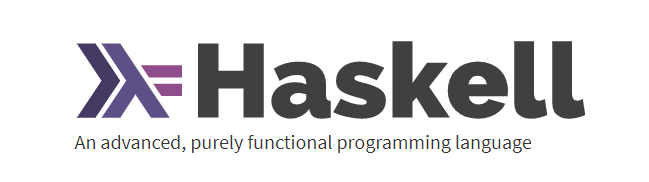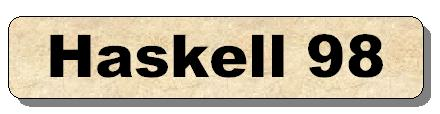## latest edition： 21/12/2019

The Haskell 98 Language Reportwww.haskell.org## 一丶Type classes(从入门到入门）

1. Eq 包含可判断相等性的类型。提供实现的函数是 == 和 /=。所以，只

`elem`函数的型别为:`(Eq a)=>a->[a]->Bool`。这是它在检测值是否存在于一个 List 时使用到了==的缘故。
``````ghci> 5 == 5
True
ghci> 5 /= 5
False
ghci> 'a' == 'a'
True
ghci> "Ho Ho" == "Ho Ho"
True
ghci> 3.432 == 3.432
True ``````

2. Ord 包含可比较大小的类型。除了函数以外，我们目前所谈到的所有

``````ghci> :t (>)
(>) :: (Ord a) => a -> a -> Bool

True
LT
ghci> 5 >= 2
True
ghci> 5 `compare` 3
GT  ``````

3. Show 的成员为可用字符串表示的类型。目前为止，除函数以外的所有

``````ghci> show 3
"3"
ghci> show 5.334
"5.334"
ghci> show True
"True"  ``````

``````ghci> :t read

True
12.0
3
[1,2,3,4,3]

5
5.0
ghci> (read "5" :: Float) * 4
20.0
[1,2,3,4]
ghci> read "(3, 'a')" :: (Int, Char)
(3, 'a')  ``````

5. Enum 的成员都是连续的类型 – 也就是可枚举。Enum 类存在的主要

(successer) 和前置子 (predecesor)，分别可以通过 succ 函数和 pred 函数得

Float 和 Double。

``````ghci> ['a'..'e']
"abcde"
ghci> [LT .. GT]
[LT,EQ,GT]
ghci> [3 .. 5]
[3,4,5]
ghci> succ 'B'
'C'  ``````

6. Bounded 的成员都有一个上限和下限。

``````minBound 和 maxBound 函数很有趣，它们的型别都是 (Bounded a) => a。可以说，它们都是多态常量。

ghci> minBound :: Int
-2147483648
ghci> maxBound :: Char
'\1114111'
ghci> maxBound :: Bool
True
ghci> minBound :: Bool
False  ``````

7.Num 是表示数字的 Typeclass，它的成员型别都具有数字的特征。检查一个数字的型别：

``````ghci> :t 20
20 :: (Num t) => t``````

``````ghci> :t (*)
(*) :: (Num a) => a -> a -> a``````

8. Integral 同样是表示数字的 Typeclass。Num 包含所有的数字：实数和

9. Floating 仅包含浮点类型：Float 和 Double。

## 从这里开始，你可以回归本节

``````ghci> :t (==)
(==) :: (Eq a) => a -> a -> Bool``````这个符号在这里代表了类型约束（符号左边的东西是限制）！！！！正常情况下写函数不需要你去额外加上这些类型约束，不但除了对理解没啥帮助外还会很容易出Bug（写错了）

``data Bool = False | True``

``data Maybe a = Nothing | Just a``

``````type Score = Int
data Study =  Bad Int | Normal Int| Good Int| Excellent Int deriving(Eq,Show)

| x >= 90           = Excellent x
| x >= 70 && x < 90 = Good x
| x >= 60 && x < 70 = Normal x是一个更神奇的东西，我们知道可以用来创建自己的类型，而type是可以声明之前已经创建过的类型(可以是basic types，也可以是你用data定义过的类型，一定要已经存在了才可以用type)（提高可读性）。千万不要混淆了他们两个，我在这边给一个网址type data newtype的区别希望大家好好看看。newtype的话我就先不说了，因为本身用的比较少，我这边也没有教过适用的方法，上面的资料算一个扩充吧

``type String = [Char]``

``data Shape = Circle Float Float Float | Rectangle Float Float Float Float``

## （举栗）

``````class Judge a where
judge :: a -> a -> Bool
instance Judge Bool where
judge True True = True
judge False False = True
judge _ _ = False ``````

Judge是被定义的typeclass，a是形态参数。之后你会定义他的具体形态。如果你开始检验judge的形态，他会受到自己这个类的影响限制, Judge（就当做自己写一个函数了，judge）

## 另外一个

``````class Comp a where
comp :: a -> a -> Integer
instance Comp Integer where
comp x y = x - y

data Circle = Circle Integer String

instance Comp Circle where
comp (Circle r1 _) (Circle r2 _) = r1 - r2

instance Show Circle where
Show ( Circle r colour) = "Circle radius:" ++ show r ++ "  colour": ++ show colour ``````